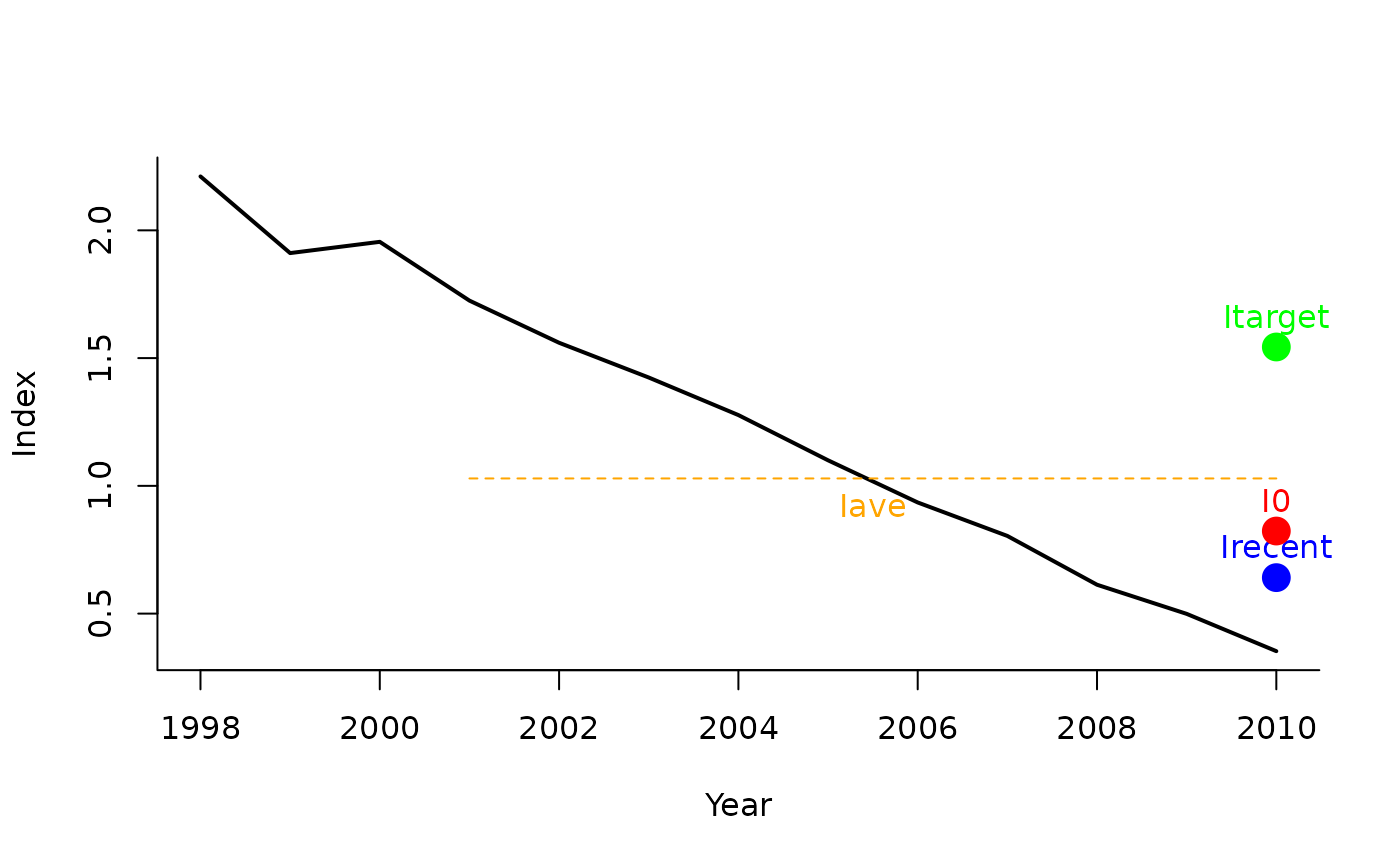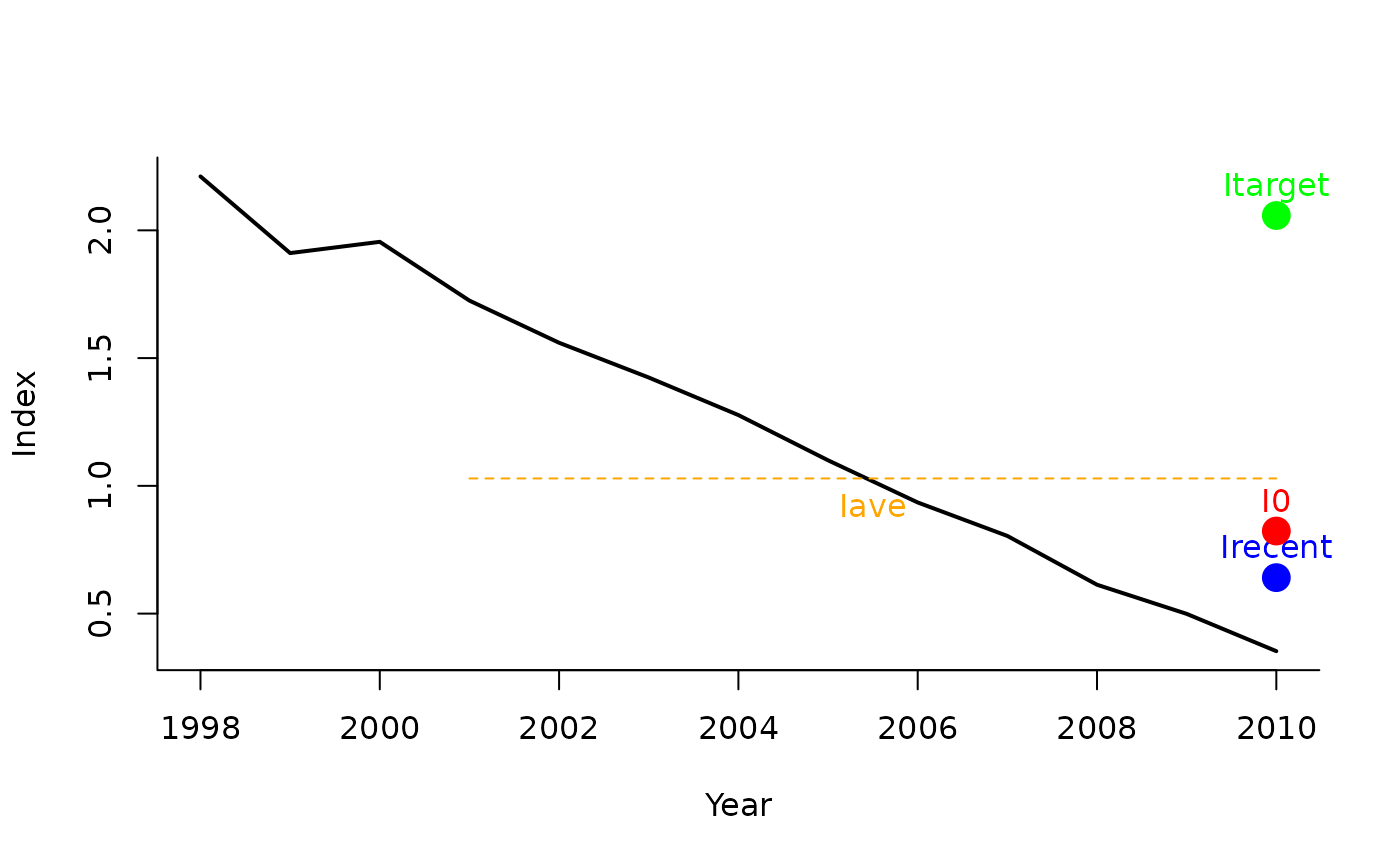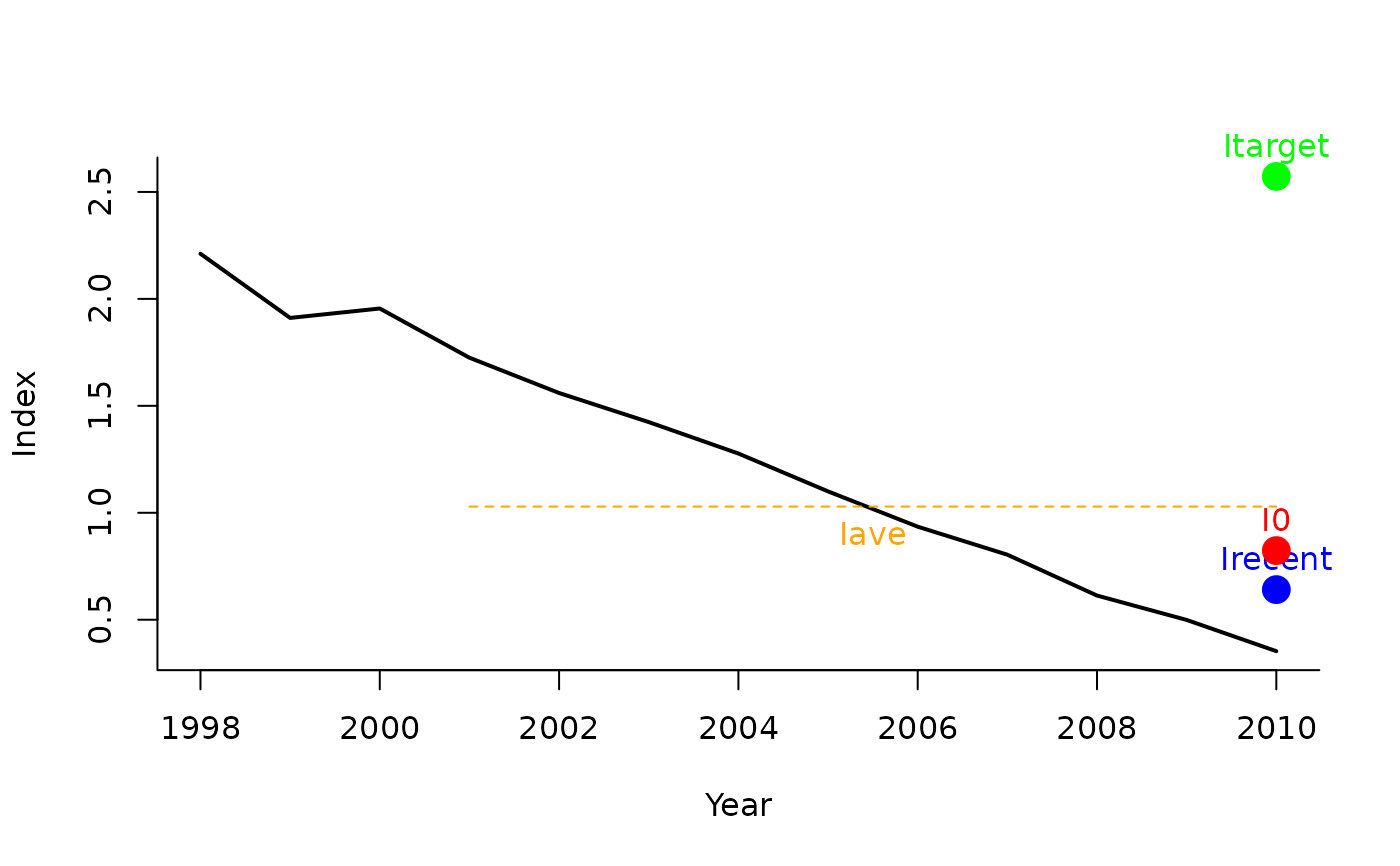A management procedure that incrementally adjusts the fishing effort to reach a target CPUE / relative abundance index

ItargetE1(x, Data, reps = 100, plot = FALSE, yrsmth = 5, Imulti = 1.5)

ItargetE2(x, Data, reps = 100, plot = FALSE, yrsmth = 5, Imulti = 2)

ItargetE3(x, Data, reps = 100, plot = FALSE, yrsmth = 5, Imulti = 2.5)

ItargetE4(x, Data, reps = 100, plot = FALSE, yrsmth = 5, Imulti = 2.5)

## Arguments

x

A position in the data object

Data

A data object

reps

The number of stochastic samples of the MP recommendation(s)

plot

Logical. Show the plot?

yrsmth

Years over which the average index is calculated.

Imulti

Parameter controlling how much larger target CPUE / index is compared with recent levels.

## Value

An object of class Rec-class with the TAE slot(s) populated

## Details

Four index/CPUE target MPs proposed by Geromont and Butterworth 2014.

The TAE is calculated as: If $$I_\textrm{recent} \geq I_0$$: $$\textrm{TAE}_y = 0.5 \textrm{TAE}_{y-1} \left[1+ \left( \frac{I_{\textrm{recent}} - I_0}{I_{\textrm{target}} - I_0} \right)\right]$$

else: $$\textrm{TAE}_y= 0.5 \textrm{TAE}_{y-1} \left( \frac{I_{\textrm{recent}}}{I_0}^2 \right)$$

where $$I_0$$ is $$0.8 I_{\textrm{ave}}$$ (the average index over the 2 x yrsmth years prior to the projection period), $$I_\textrm{recent}$$ is the average index over the past yrsmth years, and $$I_\textrm{target}$$ is Imulti times $$I_{\textrm{ave}}$$.

## Functions

• ItargetE1: The less precautionary TAE-based MP

• ItargetE2: Increasing biologically precautionary TAE-based MP

• ItargetE3: Increasing biologically precautionary TAE-based MP

• ItargetE4: The most biologically precautionary TAE-based MP

## Required Data

See Data-class for information on the Data object

ItargetE1: Ind, LHYear, MPeff, Year

## Rendered Equations

See Online Documentation for correctly rendered equations

Other Index methods: GB_slope(), GB_target(), Gcontrol(), ICI(), Iratio(), Islope1(), Itarget1_MPA(), Itarget1()

T. Carruthers

## Examples

ItargetE1(1, MSEtool::Atlantic_mackerel, plot=TRUE)#> Effort
#>   0.85
ItargetE2(1, MSEtool::Atlantic_mackerel, plot=TRUE)#> Effort
#>   0.85
ItargetE3(1, MSEtool::Atlantic_mackerel, plot=TRUE)
#> Effort
#>   0.85
ItargetE4(1, MSEtool::Atlantic_mackerel, plot=TRUE)#> Effort
#>   0.85